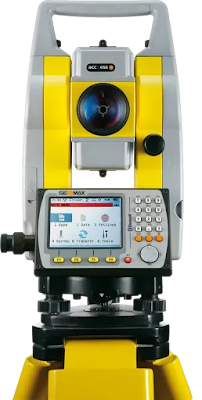What is the electromagnetic distance measurement in surveying?

# What is the electromagnetic distance measurement in surveying?

## Introduction

In this article I discussing the distance measure by electromagnetic method. In this method, the distance Is measure by the use of electromagnetic wave.
• Wave:- A wave is a disturbance that travels through a medium from one location to another location.
• Electromagnetic waves:- That wave produced a vibration between the electric field and magnetic field and has nature is perpendicular to each other.
• Wavelength:- Wavelength is defined as the distance between two successive crests or troughs of a wave.
• Frequency:- Frequency is the number of occurrence of a repeating event per unit of time.
• The wavelength of the wave:-  Speed of the wave/Frequency

In the  long-distance, the electromagnetic measurement modulation process is used. In this process measuring wave is superimposed on a very much higher frequency wave which acts as a medium for transporting the measuring wave. At the receiver, the reverse process of demodulation separates the measuring wave from the carrier wave and the phase of the received wave is converted into distance.

Generally, two types of waves are used in EDM.
1. Visible and infrared light. It has a wavelength from 450 1000 nm
or 6.7*10to 3* 105    GHz.
2. Radio waves or microwaves. It has an ave length of 35 to 3 GHz

1. Visible and infrared light:- In this method modulated infrared waves are used. prism reflectors are used at the end of the measurement line. the instrument is mounted on the theodolite. it has accuracy +- 10 mm. the range of measurement is up to 3 km.
Ex. Geodimeter, Mekometer, Rangemaster.EDM

2. Radio waves or microwaves:- It consists of two identical units. one unit is used as a master unit and the other as a remote unit. It is measured from 20 m to 150 m.
example:- Tellarometer, Distomat,

• Both are works on same as phase comparison principle to determine distance. Difference in phase between the transmitted and received waves.
Any E.D.M equipment performs the following basic functions.
• The generation of the carrier wave and measuring wave.
• The modulation and demodulation of the carrier wave.
• The measurement of the phase difference between the transmitted and received measuring waves.
• The display, in some form of the result of this measurement.

SEE ALSO:-The Use of 3D Laser scaning Technology in Mining and Surveying

What Is The Gps Survey And used in mine surveying